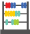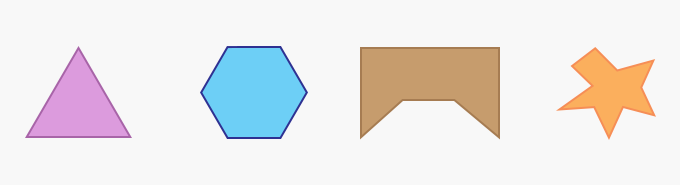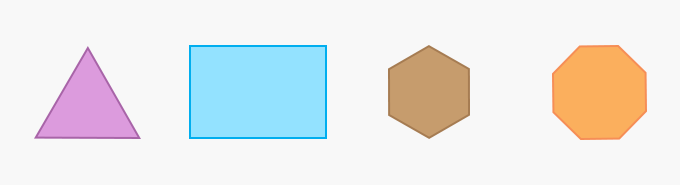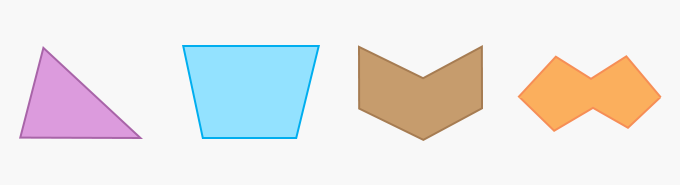# Polygon - Definition with Examples

The Complete K-5 Math Learning Program Built for Your Child

• 30 Million Kids

Loved by kids and parent worldwide

• 50,000 Schools

Trusted by teachers across schools

• Comprehensive Curriculum

Aligned to Common Core

##Let's learn!

What is a polygon?
In geometry, a polygon can be defined as a flat or plane, two-dimensional closed shape with straight sides. It does not have curved sides.

Here are a few examples of polygons.Polygons can be of two types:

Regular Polygons – Polygons that have equal sides and angles are regular polygons.

Here are a few examples of regular polygons.Irregular Polygons – Polygons with unequal sides and angles are irregular polygons.

Here are a few examples of irregular polygons.Fun Facts Quadrilaterals are also polygons.

##Let's sing!

All Polygons are closed figures,
As count of sides gets bigger,
The names of polygons differ!

##Let's do it!

Show your child pictures of different polygons such as a triangle, rectangle, pentagon and hexagon. Ask them to count the number of sides each have and tell them its name. You can further explain how these polygons have been named based on the number of sides they have.

##Related math vocabulary

Won Numerous Awards & Honors